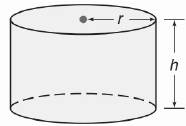Chapter 9.CT, Problem 12CTElementary Geometry For College St...

7th Edition
Alexander + 2 others
ISBN: 9781337614085

Solutions

Chapter
SectionElementary Geometry For College St...

7th Edition
Alexander + 2 others
ISBN: 9781337614085
Textbook Problem

For the right circular cylinder shown, r = 4 cm and h = 6 cm. Find the exacta) lateral area.b) volume.

To determine

a)

To find:

The exact lateral area of the right circular cylinder.

Explanation

Given:

For the right circular cylinder r=4 cm, h=6 cm

Formula used:

The lateral area of the right circular cylinder is L=2πrh.

Calculation:

We have r=4,h=6

Substitute the known values in L=2πrh

To determine

b)

To find:

The exact volume of the right circular cylinder.

Still sussing out bartleby?

Check out a sample textbook solution.

See a sample solution

The Solution to Your Study Problems

Bartleby provides explanations to thousands of textbook problems written by our experts, many with advanced degrees!

Get Started

Simplify: 912

Elementary Technical Mathematics

In problems 17-20, indicate whether the two sets are equal. 18.

Mathematical Applications for the Management, Life, and Social Sciences

Expand each expression in Exercises 122. (x+1)(x3)

Finite Mathematics and Applied Calculus (MindTap Course List)

Write the following numbers in word form. .00938

Contemporary Mathematics for Business & Consumers

In Exercises 918, indicate whether the matrix is in row-reduced form. [100100|1020]

Finite Mathematics for the Managerial, Life, and Social Sciences